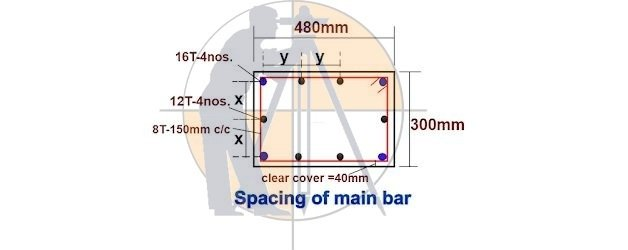Breaking News
Home / Civil Engineering / Bar Bending Schedule / Calculate Center To Center Distance Between The Bars In Column,Beam And slab

# Calculate Center To Center Distance Between The Bars In Column,Beam And slab

## Calculate Center To Center Distance Between The Bars In Column – Beam And slab

Let us calculate the C / C distance between the reinforcement bars for the column section drawing as shown below.### Given data :

Column width = 480mm.

Column depth = 300mm.

Clear cover = 40mm.

Corner rebar diameter = 16mm.

Mid rebar diameter = 12mm.

No. of rebars = 10nos.

Stirrup bar diameter = 8mm.

2. ### Bar Bending Schedule Of Lintel Beam With Full Detail

Now, we will find out the spacing of the bars in both directions, i.e. the value of x & y as shown in the drawing.

First, we will calculate where,

y = [column width – {(2nos.× cover) +( 2nos.× dia. of stirrup bar ) +(2nos.×1/2 dia. of corner bar)}] ÷[ no. of bars in width side -1]

= [ 480mm – {( 2nos. × 40mm ) + ( 2nos. × 8mm ) + ( 2nos. × 1/2 × 16mm )}] ÷ [4 -1]

=  [ 480mm – { 80mm + 16mm + 16mm }] ÷ 3

= [ 480mm – 112mm ] ÷ 3

= 368mm ÷ 3

### Bar Bending Schedule Of Plinth Beam

= [column depth – {(2nos.× cover) +( 2nos.× dia. of stirrup bar ) +(2nos.×1/2 dia. of corner bar)}] ÷[ no. of bars in depth side -1]

= [ 300mm – {( 2nos. × 40mm ) + ( 2nos. × 8mm ) + ( 2nos. × 1/2 × 16mm )}] ÷ [3 -1]

=  [ 300mm – { 80mm + 16mm + 16mm }] ÷ 2

= [ 300mm – 112mm ] ÷ 2

= 188mm ÷ 2

### = 94mm.

The center-to-center distance of bars in the column is y = 122.66mm. & x = 94mm.

### Note:

1. By using this method, you can calculate the center-to-center distance between all types of structural members.

2. The center-to-center distance will be the same irrespective of the diameter of bars.

### THANKS.